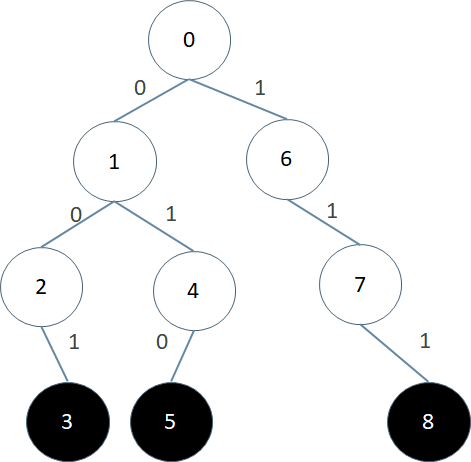# TRIE（3）

## July 7, 2018 • Read: 347 • 算法

#### 例2 题目链接：hihoCoder1107Insert(W):
P = root
Cnt[p]++
For i = 1...W.len
If p.thru(W[i]) == NULL//没有标识为W[i]的边
P = P.thru(W[i])
Cnt[p]++
P.markEndPoint()//标记P为终结点
DFS(P,father):
If Cnt(p) <= 5 AND Cnt(father) > 5
Ans++
Return
For i = 0...25
If P.thru(i) != 0
DFS(P.thru(i),p)
Main:
For i = 0...N-1
Insert(S[i])
Ans = 0
DFS(root,-1)
Print ANS

完整的代码如下：

#include <cstdio>
#include <cstring>
using namespace std;
const int MAX_NODE = 1000000 + 10;
const int CHARSET = 26;
int trie[MAX_NODE][CHARSET] = {0};
int cnt[MAX_NODE] = {0};
int n,ans,k = 1;
char s;
void insert(char *w) {
int len = strlen(w);
int p = 0;
cnt++;
for(int i = 0;i < len;i++) {
int c = w[i] - 'a';
if(!trie[p][c]) {
trie[p][c] = k;
k++;
}
p = trie[p][c];
cnt[p]++;
}
}
void dfs(int x,int father) {
if(x != 0 && cnt[x] <= 5 && cnt[father] > 5)
ans++;
for(int i = 0;i < 26;i++)
if(trie[x][i])
dfs(trie[x][i],x);
}
int main() {
memset(trie,0,sizeof(trie));
scanf("%d",&n);
for(int i = 0;i < n;i++) {
scanf("%s",s);
insert(s);
}
dfs(0,-1);
printf("%d\n",ans);
return 0;
}

For i = 1...M
Ans = 0
For j = 1...N
If A[j] xor x > Ans xor x
Ans = A[j]
Print A[j]所以我们第一步沿着标识是0的边，移动到了1号节点；4第二位是0，所以我们沿着标识是1的边移动到4号节点；4的第三位是0，但是4号节点没有标识是1的边，所以我们也只好沿着标识是0的边移动到5号节点。已经到了终结点，所以5号节点对应的A2=(010)2=2就是我们要求的答案，A2 xor 4 = 6是最大的

下面我们不忙写代码，我们先再看一道进阶版的题目：给定一个包含N个整数的数组A=[A1, A2, A3, … AN]，让你求出一段连续的子数组A[l], A[l+1], … A[r]使得异或和A[l] xor A[l+1] xor A[l+2] xor … xor A[r]的值最大

解决这道题目的关键，就是利用之前我们在枚举中提到的一个技巧，就是用前缀和表示部分和。之前我们遇到的部分和题目是加法的和，这里异或的和其实是一样的。我们定义S[i]=A xor A xor A xor … xor A[i]，那么A[l] xor A[l+1] xor A[l+2] xor … xor A[r]就等于S[r] xor S[l-1]。因为两个相同的数异或起来值是0

所以这道题就变成了下面这样：给定一个数组：A=[A1, A2, … AN]，我们先求出对应的xor前缀和S, S, … S[N]，问题是从S数组中找出两个数使得它们的异或值最大。这时就可以用到上一道题的方法了，伪代码如下：

S = 0
Ans = 0
Trie.insert(0)
For i = 1...N
S = S xor A[i]
T = Trie.search(S)
Ans = max(Ans,T)
Tire.insert(S)
Print(Ans)

完整的代码如下：

#include <cstdio>
#include <cstring>
using namespace std;
const int MAX_NODE = 1000000 + 10;
const int CHARSET = 2;
int trie[MAX_NODE][CHARSET] = {0};
int color[MAX_NODE] = {0},a;
int t,n,m,ans,k = 1,b;
void insert(int x) {
for(int i = 31;i >= 0;i--) {
b[i] = x % i;
x >>= 1;
}
int p = 0;
for(int i 0;i < 32;i++) {
int c = b[i];
if(!trie[p][c]) {
trie[p][c] = k;
k++;
}
p = trie[p][c];
}
color[p] = 1;
}
int search(int x,int father) {
int ret = 0;
for(int i = 31;i >= 0;i--) {
b[i] = x %  i;
x >> =1;
}
int p = 0;
for(int i 0;i < 32;i++) {
int c = 1 - b[i];
if(!trie[p][c]) {
p = trie[p][1 - c];
ret *= 2;
}
else {
p = trie[p][c];
ret = ret * 2 + 1;
}
}
return ret;
}
int main() {
scanf("%d",&t);
while(t--) {
memset(trie,0,sizeof(trie));
memset(color,0,sizeof(color));
insert(0);
ans = 0;
int s = 0;
scanf("%d",&n);
for(int i = 0;i < n;i++) {
scanf("%d",&a[i]);
s = s ^ a[i];
int tmp = search(s);
ans = max(ans,tmp);
insert(s);
}
printf("%d\n",ans);
}
return 0;
}

第9~24行是insert函数，这里我们插入一个整数x，先把x对应的二进制01串求出来，b是最高位，b是最低位。然后再把b..b按位插入到trie中

第25~44行是search函数，注意我们要尽量与b[i]反着走，也就是尽量沿着c=1-b[i]走。如果trie[p][c]不存在，那么意味着这一位的异或值只能是0了，即ret = ret * 2；否则以为这一位异或可以是1，即ret = ret * 2 + 1